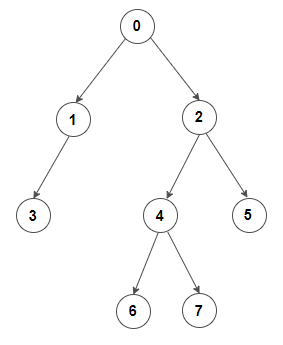# Build a binary tree from a parent arrayGiven an integer array representing a binary tree, such that the parent-child relationship is defined by `(A[i], i)` for every index `i` in array `A`, build a binary tree out of it. The root node’s value is `i` if `-1` is present at index `i` in the array. It may be assumed that the input provided to the program is valid.

For example,

Parent: [-1, 0, 0, 1, 2, 2, 4, 4]
Index : [ 0, 1, 2, 3, 4, 5, 6, 7]

Note that,

• -1 is present at index 0, which implies that the binary tree root is node 0.
• 0 is present at index 1 and 2, which implies that the left and right children of node 0 are 1 and 2.
• 1 is present at index 3, which implies that the left or the right child of node 1 is 3.
• 2 is present at index 4 and 5, which implies that the left and right children of node 2 are 4 and 5.
• 4 is present at index 6 and 7, which implies that the left and right children of node 4 are 6 and 7.

The corresponding binary tree is:Practice this problem

The solution is simple and effective – create `n` new tree nodes, each having values from 0 to `n-1`, where `n` is the array’s size, and store them in a map or array for the quick lookup. Then traverse the given parent array and build the tree by setting the parent-child relationship defined by `(A[i], i)` for every index `i` in array `A`. Since several binary trees can be formed from a single input, the solution should build any of them. The solution will always set the left child for a node before setting its right child.

The algorithm can be implemented as follows in C++, Java, and Python:

Output:

3 1 0 6 4 7 2 5

Output:

3 1 0 6 4 7 2 5

## Python

Output:

3 1 0 6 4 7 2 5

The time complexity of the above solution is O(n), where `n` is the total number of nodes in a binary tree (assuming constant-time operations for the hash table). The auxiliary space required by the program is O(n).

Rate this post

Average rating 4.77/5. Vote count: 176

No votes so far! Be the first to rate this post.

We are sorry that this post was not useful for you!

Tell us how we can improve this post?